# Train speed

Two guns were fired from the same place at an interval of 10 minutes and 30 seconds, but a person in a train approaching the place hears second shot 10 minutes after the first. The speed of the train (in km/hr), supposing that sound travels at 340 m/s is:

v =  61.2 km/h

### Step-by-step explanation: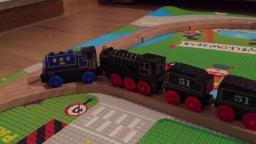Did you find an error or inaccuracy? Feel free to write us. Thank you!Tips to related online calculators
Do you have a linear equation or system of equations and looking for its solution? Or do you have a quadratic equation?
Do you want to convert length units?
Do you want to convert velocity (speed) units?
Do you want to convert time units like minutes to seconds?

## Related math problems and questions:

• The shooter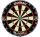The shooter heard the impact of the bullet on the target in one second after the shot. The bullet was moving at an average speed of 500 m/s. Calculate with speed of sound of 340 m/s. Determine the distance of the target.
• Sound speed in Earth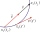Through the surface layers of the Earth, sound propagates 13 times faster than through air. During the geological survey, the charge exploded 1.7 km from the measuring station. How long does it take between the vibration of the soil at the measuring point
• TrainsFrom station 130 km away started passenger train and after 2.2 hours after the express train, which travels 37 km an hour more. Express train finish journey 7 minutes early. Calculate the average speed of this two trains.
• Speed of sound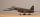The average speed of sound is 330 meters per second. Estimate how long it will take to hear the church bell 1 km away. Calculate the distance from what would hear sound after 10 seconds.
• The train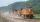The train passed the observer in the 20s and passed through a 1500m tunnel in 2 minutes. Calculate the speed (km/h) and train length.
• Two trainsThrough the bridge, long l = 240m, the train passes through the constant speed at time t1 = 21s. A train running along the traffic lights at the edge of the bridge passes the same speed at t2 = 9s. a) What speed v did the train go? b) How long did it take
• Train speed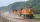The train speed is decreased during 50 sec from 72 km/h to 36 km/h. Assuming that the train movement is equally slowing, find the the acceleration and the distance that it travels at.
• Bus vs. trainThe bus started from point A 10 minutes before the train started from the same place. The bus went an average speed of 49 km/h, train 77 km/h. To point B train and bus arrived simultaneously. Calculate the time of the train journey, if train and bus trave
• Two trainsThe train runs at speed v1 = 72 km/h. The passenger, sitting in the train, observed that a train long l = 75m in 3 s passed on the other track in the opposite direction. Calculate the speed of this train.
• Ascent and descent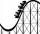Car goes 114 km track, which consists of ascent and descent at time of 1 hour 35 minutes. When climbing moves at the speed 48 km/h and downhill 25 m/s. What is the length of climb and descent?
• Runner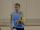Peter ran a speed of 12.4 km / h. After 45 minutes running he had a hiatus. The track is 31 km long. How many kilometers he still to run if he still running at the same speed?
• Speed of Slovakian trainsRudolf decided to take the train from the station 'Ostratice' to 'Horné Ozorovce'. In the train timetables found train Os 5409 : km 0 Chynorany 15:17 5 Ostratice 15:23 15:23 8 Rybany 15:27 15:27 10 Dolné Naštice 15:31 15:31 14 Bánovce nad Bebravou 15:35 1
• Train and car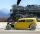The train and the car started at a constant speed to journey. When the train travels 87 km, the car travels 97 km. How many km travels the train when the car travels 87 km?
• Double-track line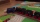A 160 m long passenger train runs on a double-track line in one direction at a constant speed of 54 km/h, and a 240 m long express train in the opposite direction. a) How fast is the express train if passing the passenger train driver for 6 s? b) How long
• Motion 2At 10 o'clock started from place A car speed at 90 km/h. 20 minutes after started from the place B at speed 60 km/h another car. Distance places A and B is 120 kilometers. What time and where they meet?
• Two trains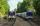Two trains go at each other. First at speed 40 km/h and the second at speed 60 km/h. At what distance will be 30 minutes before they will meet?
• Two trains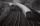There are two trains running the same distance. 1st train will travel it in 7 hours 21minutes. 2nd the train will travel 5 hours 57minutes and 34 seconds and it is 14 km/h faster than the first train. What are speeds of trains and how long is this railway